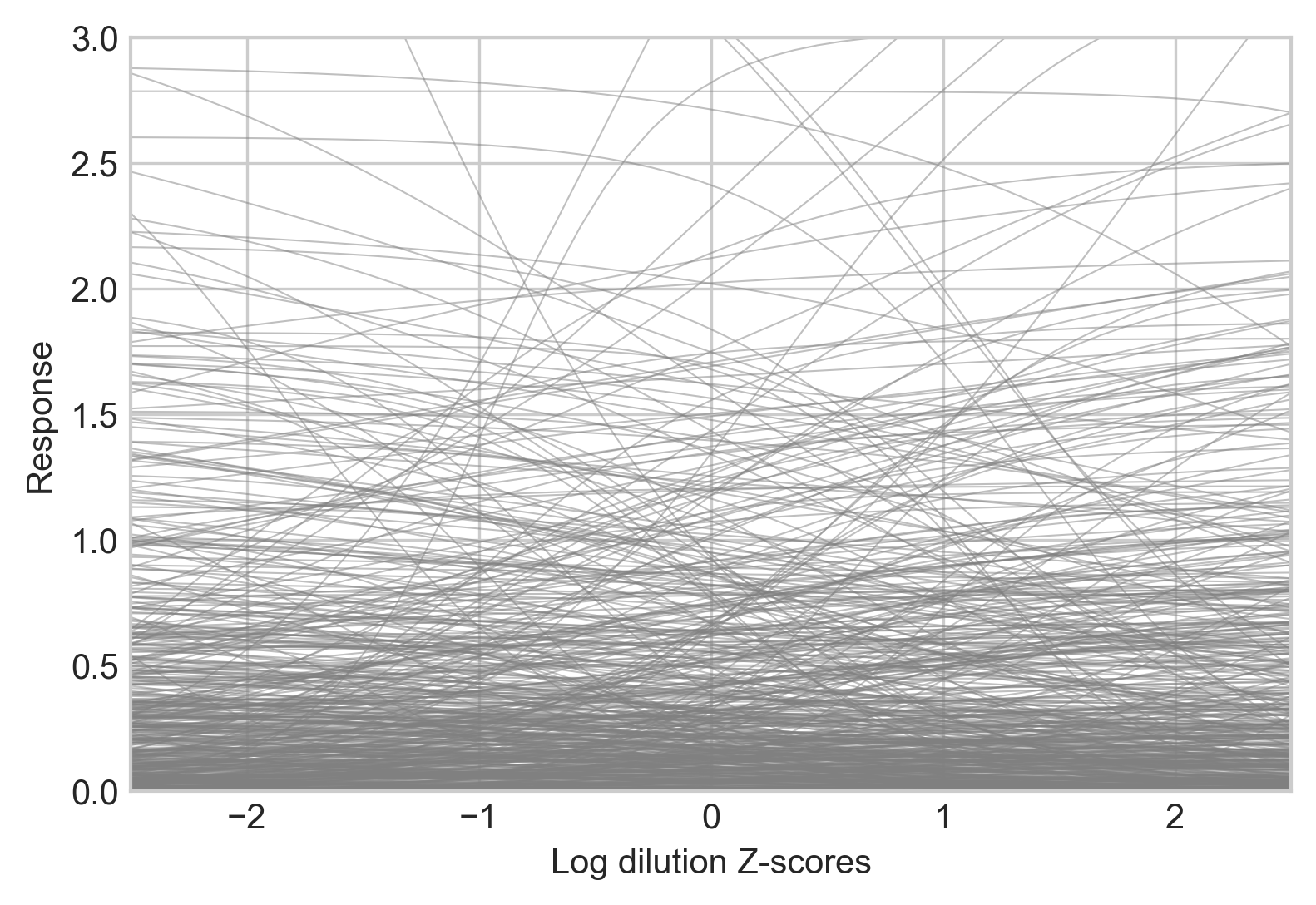# Bayesian Inference¶

ititer uses Bayesian inference to infer posterior distributions of sigmoid curve parameters.

Formally, the response of sample i, $$y_i$$, is modelled as a function of log dilution using a four parameter logistic curve, $$x_i$$:

\begin{align}\begin{aligned}\mu_i = c + \frac{d}{1 + e^{-b(x_i - a)}}\\\sigma \sim \text{dexp}(1)\\y_i \sim \text{dnorm}(\mu_i, \sigma)\end{aligned}\end{align}

Parameters are interpreted as follows:

• $$a$$ - horizontal location of the sigmoid

• $$b$$ - gradient at the inflection point

• $$c$$ - minimum response

• $$d$$ - difference between minimum and maximum

Parameters can either be set a priori, inferred using full pooling (yielding a single distribution from all samples), or inferred using partial pooling (where each sample gets its own posterior distribution).

## Standardizing¶

Log dilution is standardized to have mean of zero and standard deviation of one for inference.

Response is standardized to have a minimum of 0 and maximum of 1.

## Priors¶

Weakly informative priors are used by default throughout. When parameters a, b, and c are fully pooled, they given a prior of $$\text{dnorm}(0, 1)$$. When a, b, and c are partially pooled across samples, s, they are given priors of:

\begin{align}\begin{aligned}\mu_p \sim \text{dnorm}(0, 1)\\\sigma_p \sim \text{dexp}(1)\\p_s \sim \text{dnorm}(\mu_p, \sigma_p)\end{aligned}\end{align}

To prevent redundancy between the values of c and d, d is constrained to be positive. When it is fully pooled: $$d \sim \text{dexp}(1)$$. When it is partially pooled:

\begin{align}\begin{aligned}\sigma_d \sim \text{dexp}(1)\\d_s \sim \text{dexp}(\sigma_d)\end{aligned}\end{align}

These priors will be applicable for most users.

Partial pooling for a, full pooling for b and d, and setting c=0 gives the following prior predictive distribution:Code used to generate this figure:

import numpy as np
import matplotlib.pyplot as plt
import ititer as it

df["Log Dilution"] = it.titer_to_index(df["Dilution"], start=40, fold=4)

sigmoid = it.Sigmoid().fit(
data=df,
response="OD",
sample_labels="Sample",
log_dilution="Log Dilution",
prior_predictive=True,
)

x = np.linspace(-2.5, 2.5).reshape(-1, 1)
y = it.inverse_logit(
x=x,
a=sigmoid.prior_predictive["a"][:, 0],
b=sigmoid.prior_predictive["b"],
c=0,
d=sigmoid.prior_predictive["d"],
)

plt.plot(x, y, c="grey", alpha=0.5, lw=0.5)
plt.xlabel("Log dilution Z-scores")
plt.ylabel("Response")
plt.ylim(0, 3)
plt.xlim(-2.5, 2.5)
plt.savefig("prior-predictive.png", bbox_inches="tight", dpi=300)